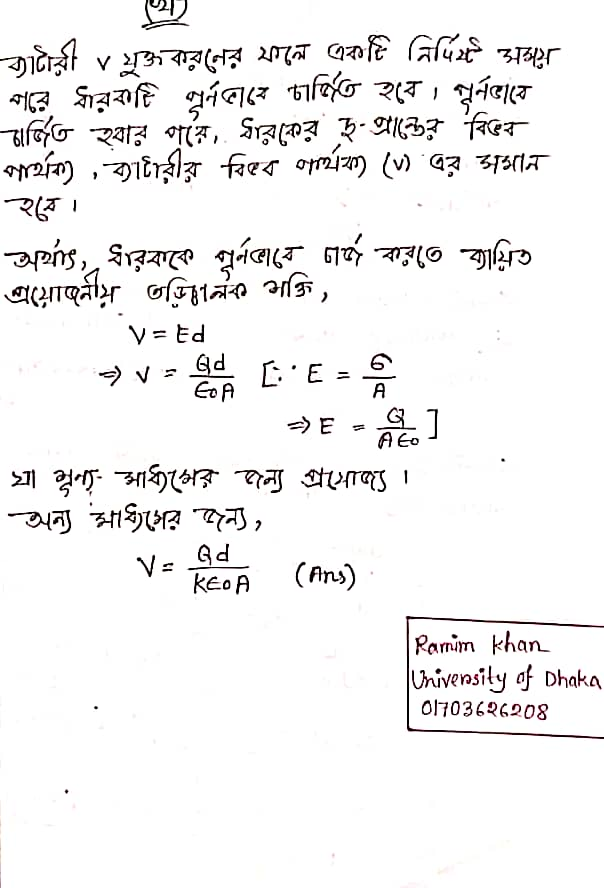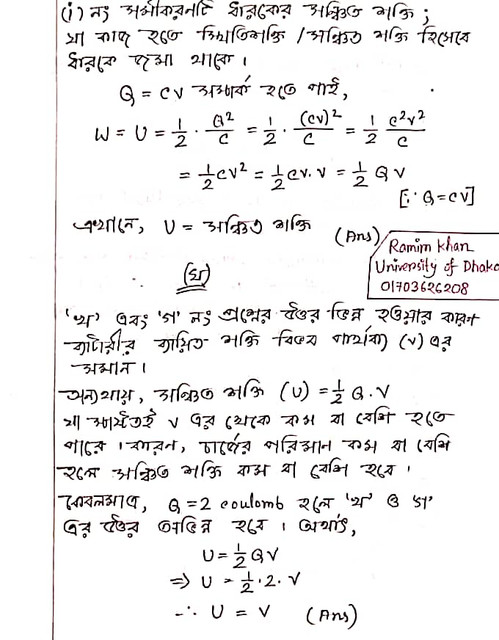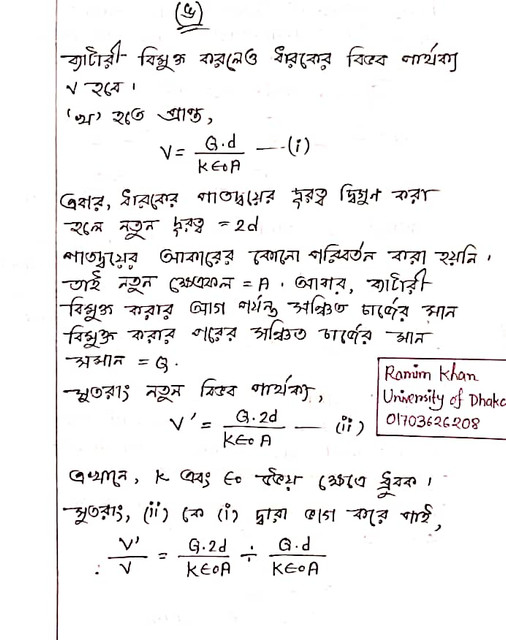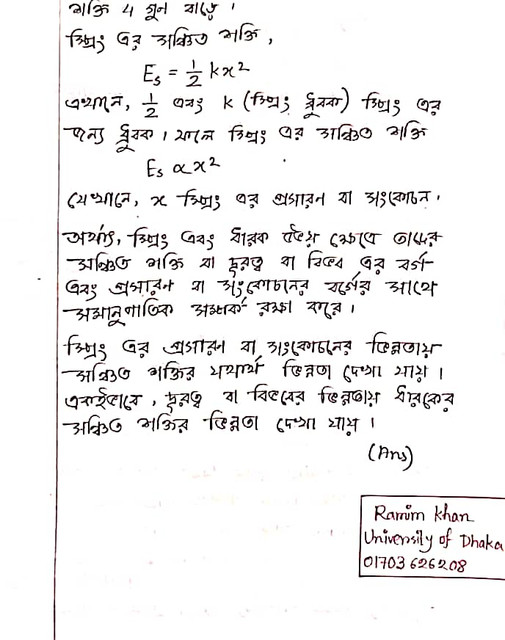Assignment

Dear students of HSC 2023 academic year, here is your desired HSC 2023 Physics 2nd Paper Assignment Solution – Problems related to holder capacity and stored energy. We will follow the instructions given in Physics 2nd Paper Assignment of HSC Exam 2023 and try to discuss the instructions mentioned in the question continuously so that it is convenient for you to write the assignment.

By practicing this discussion properly, you will be able to complete the solution / answer of the Physics 2nd Paper Assignment for the students of the seventh week of the HSC examination of 2023 for the public-private educational institutions of the country.

## HSC Physics 7th Week Assignment 2023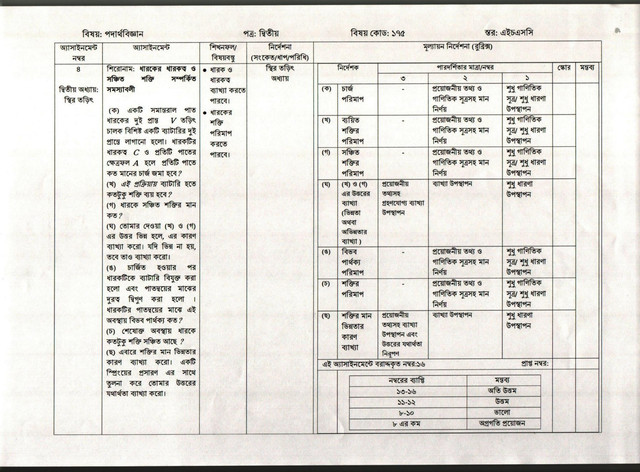Assignment: Problems related to container capacity and stored energy

(A) The two ends of a parallel sheet holder are connected to the two ends of a battery with an electric conductor. If the capacity of the container is C and the area of ​​each leaf is A, what is the value of charge per leaf?

(B) How much energy will be expended from the battery in this process?

(C) What is the value of energy stored in the container?

(D) If the answers given by Tayma (b) and (c) are different, explain the reason. If not different, then explain.

(E) After charging, the battery is disconnected from the container and the distance between the two sheets is doubled. What is the potential difference between the two leaves of the container?

(F) How much energy does the borrower have in the last stage?

(G) This time to explain the reason for the difference in the value of energy. Explaining the validity of Tayma’s answer by comparing it to the expansion of a spring.

Instruction (signal / step / circumference): static electricity section

### HSC Physics 7th Week Assignment Answer 2023